Courses

# Bipolar Junction Transistor MCQ Level -2

## 10 Questions MCQ Test Topic wise Tests for IIT JAM Physics | Bipolar Junction Transistor MCQ Level -2

Description
This mock test of Bipolar Junction Transistor MCQ Level -2 for Physics helps you for every Physics entrance exam. This contains 10 Multiple Choice Questions for Physics Bipolar Junction Transistor MCQ Level -2 (mcq) to study with solutions a complete question bank. The solved questions answers in this Bipolar Junction Transistor MCQ Level -2 quiz give you a good mix of easy questions and tough questions. Physics students definitely take this Bipolar Junction Transistor MCQ Level -2 exercise for a better result in the exam. You can find other Bipolar Junction Transistor MCQ Level -2 extra questions, long questions & short questions for Physics on EduRev as well by searching above.
QUESTION: 1

### A bipolar junction transistor with one junction forward biased and either the collector or emitter open, operates in the.

Solution:

When one junction is forward biased and either the collector or emitter is open, the BJT will operate in pinch-off region.
The correct answer is: pinch-off region

QUESTION: 2

### A common emitter transistor amplifier circuit is operated under a fixed bias. In this circuit, the operating point.

Solution:

In fixed bias, when temperature is increased, the operating point moves toward the saturation region.
The correct answer is: moves towards the saturation region with a decrease in temperature

QUESTION: 3

### In a typical npn transistor, the doping concentrations in emitter, base and collector regions are nE, nB, nC respectively. These satisfy the relation.

Solution:

In an npn transistor, emitter heavily doped, collector is moderately doped and base very thin and least doped, i.e.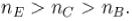The correct answer is:QUESTION: 4

If for a silicon npn transistor, the base to emitter voltage (VBE) is 0.7Vand the collector to base voltage (VCB) is 0.2V, then the transistor is operating in the

Solution:

VB = 0.7V  (Forward-biased)
and VBC = -VCB
= -0.2V
(Reverse-biased)
hence, the transistor operates in normal active mode.
The correct answer is: normal active mode

QUESTION: 5

At very high frequency, mica capacitor are used, because.

Solution:

At high frequencies, mica capacitors are used because mica has very high resistivity at these frequencies. Mica is very useful material for making the frequencial substances.
The correct answer is: mica has high resistivity at these frequencies

QUESTION: 6

A piece of semiconductor material is introduced in a circuit. If the temperature of the material is raised, the circuit current n t will decrease.

Solution:

The circuit current will increase because as temperature of the semiconductor material increases , its resistance decreases .

QUESTION: 7

For an amplifier, the base of an npn transistor must be.

Solution:

The base of an npn transistor must be positive with respect to emitter. The correct answer is: positive with respect to emitter

QUESTION: 8

Which condition will saturate the BJT.

Solution:

If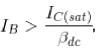the BJT will go into the state of saturation.
The correct answer is: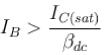QUESTION: 9

In a voltage divider biased transistor circuit such as in figure given below.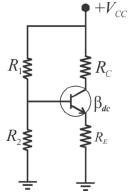Rin(base) can 9enerally t>e neglected in calculations when.

Solution:

If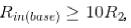then Rin (Base) can be neglected in the calculation.
The correct answer is:QUESTION: 10

Which one of the following statements is true for a semiconductor p - n junction with no-external bias?

Solution:

The region containing the uncomposed acceptor and and donor ions is called depletion region i.e., there is a depletion of mobile charge (holes and free electrons) in this region. Since, this region has immobile ions which are electrically charge, it is also referred to as the space charge region. The electrical field between the acceptor and the donor ions is called a barrier. It is clear that p-side of the junction is negatively charged.
The correct answer is: The p-side of the junction is negatively charged